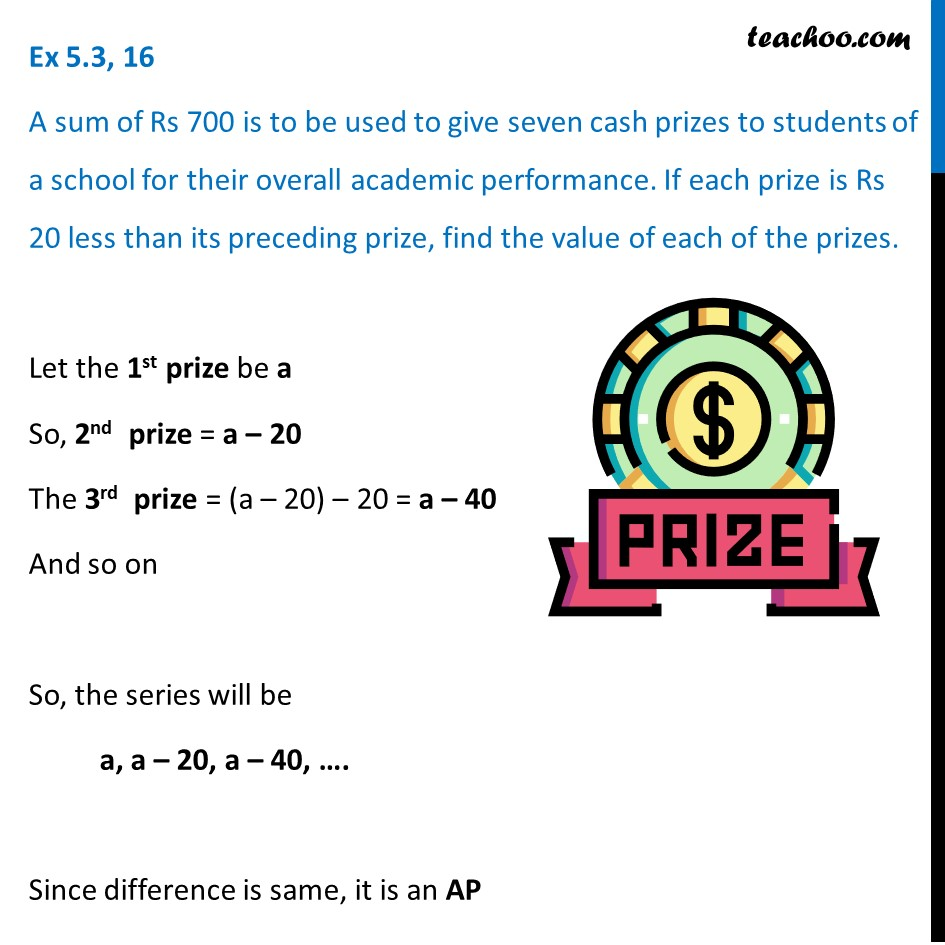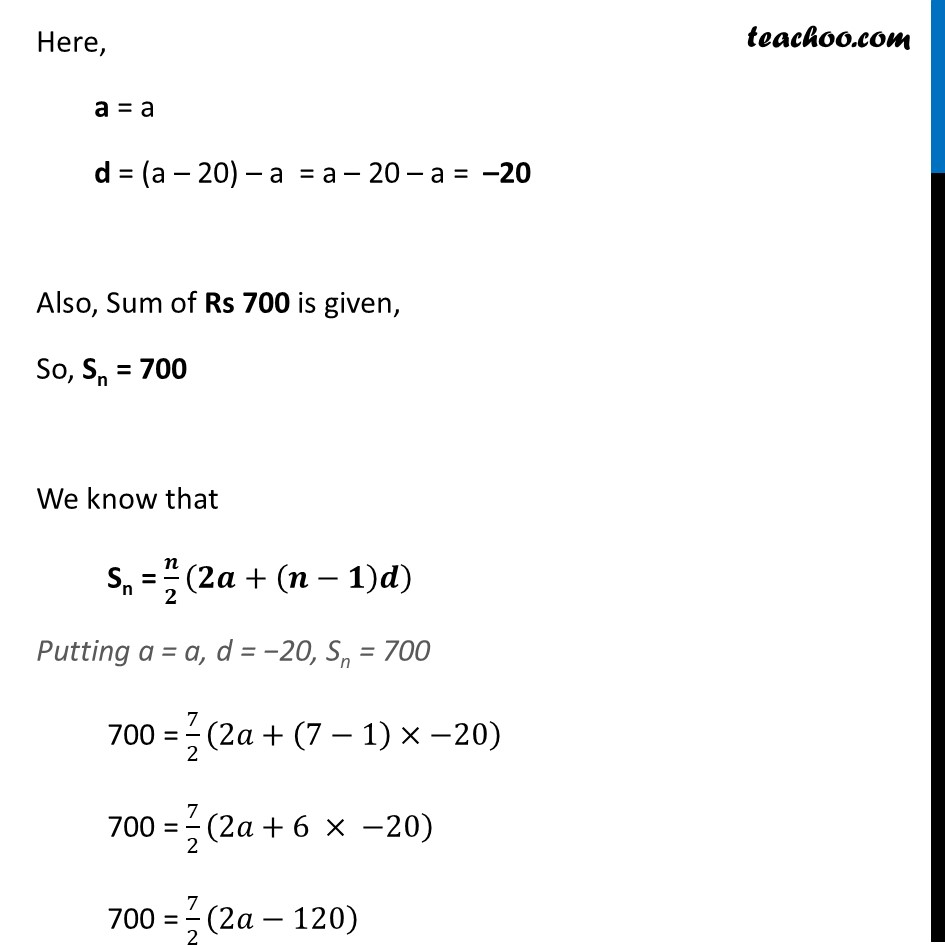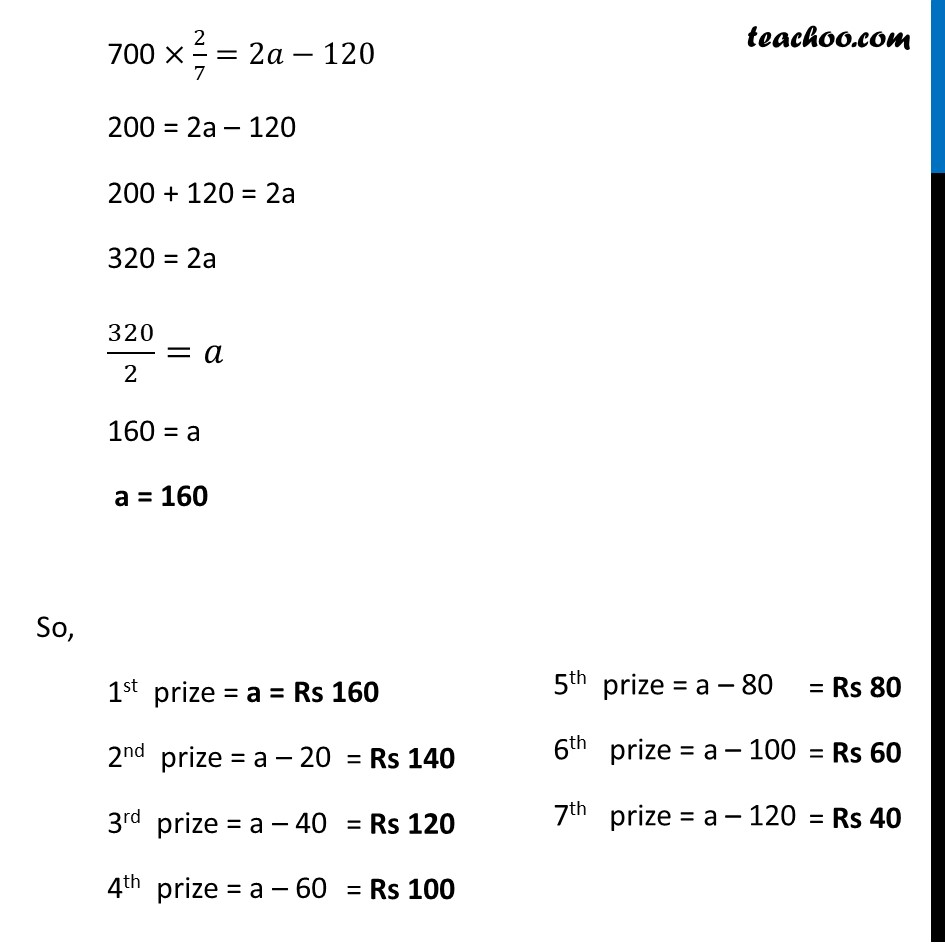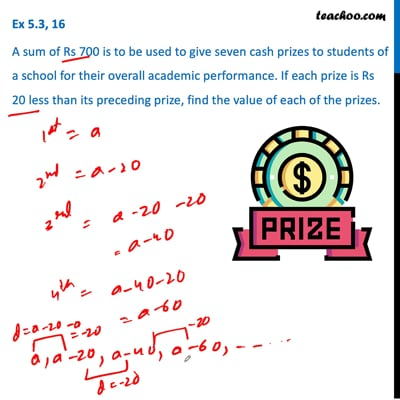Finding sum of n terms

Chapter 5 Class 10 Arithmetic Progressions (Term 2)
Concept wiseThis video is only available for Teachoo black users

### Transcript

Ex 5.3, 16 A sum of Rs 700 is to be used to give seven cash prizes to students of a school for their overall academic performance. If each prize is Rs 20 less than its preceding prize, find the value of each of the prizes. Let the 1st prize be a So, 2nd prize = a – 20 The 3rd prize = (a – 20) – 20 = a – 40 And so on So, the series will be a, a – 20, a – 40, …. Since difference is same, it is an AP Here, a = a d = (a – 20) – a = a – 20 – a = –20 Also, Sum of Rs 700 is given, So, Sn = 700 We know that Sn = 𝒏/𝟐 (𝟐𝒂+(𝒏−𝟏)𝒅) Putting a = a, d = −20, Sn = 700 700 = 7/2 (2𝑎+(7−1)×−20) 700 = 7/2 (2𝑎+6 × −20) 700 = 7/2 (2𝑎−120) 700 × 2/7=2𝑎−120 200 = 2a – 120 200 + 120 = 2a 320 = 2a 320/2=𝑎 160 = a a = 160 So, 1st prize = a = Rs 160 2nd prize = a – 20 3rd prize = a – 40 4th prize = a – 60 5th prize = a – 80 6th prize = a – 100 7th prize = a – 120